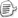POJ-1061　　拓展欧几里得的应用，需要开long long

第一次做这个题的时候进行了毫无用处的找公式（？），是个现在的我看不懂的迷之思路。

第二发的时候还是不明白位数与数据大小的年轻人，1e9以上开long long，可以到1e18，2^63以上直接ull，2^64及以上考虑一下换思路吧。

先放乱码#include<cstdio>
#include<iostream>
using namespace std;
long long r;

long long exp(long long a,long long b, long long&x,long long &y)
{
if(b==0){
x=1;
y=0;
return a;
}
r=exp(b,a%b,y,x);
y-=a/b*x;
//printf("%d\n",b);
return r;
}

int main()
{
long long x,y,m,n,L,t,c;
cin>>x>>y>>m>>n>>L;
exp(n-m,L,t,c);
if ((x-y)%r!=0) cout<<"Impossible"<<endl;
else {
t*=(x-y)/r;
c*=(x-y)/r;
t=(t%L+L)%L;
cout<<t<<endl;
}
return 0;
}

首先，同余符号：≡。然后，丢番图方程。最后，贝祖定理。好的这些都没有什么直接关系。

我们要求一个t，使得x + t*m ≡ y + t*n (mod L)，x和y是已知的位置，m和n是速度，L是一圈的大小。同余在加减法没有什么特别的，我们可以通过移项得到(n-m) * t ≡ x-y (mod L)，设倍数为c，化为等式即 （n-m）*t+L*c=x-y。现在我们得到了一个二元一次不定方程 at+bc=z。（为了方便理解我不把未知数替换成xy了）

第二个要求是获得整数k解。那么我们设r=gcd（a,b），并在方程两边除以r，那么未知数系数均为整数，解为整数，可得条件z/r为整数。不符合这个条件就Impossible。这个方程会不会无解呢？贝祖定理告诉我们ax+by=z是必定有整数解的，所以我们只要得到ax0+by0=z的解，就可以得到t=(z/r*x0),c=(z/r*y0)。（显然r*z/r=z，ok吗，不知道为什么我之前这里不ok_(:з」∠)_）

现在，我们来用拓欧算法求解x0，y0吧|ू･ω･` )

1）b==0，则x=1，y=0；

2）b！=0，gcd（a,b）=gcd（b,a%b），a%b=a-a/b*b（众所周知，自动向下取整）则ax0+by0=by1+(a-a/b*b)x1，x0=x1,y0=y1-a/b*y1（递归中我直接交换了两个未知数的位置）

拓展就在于在求gcd之外我们要在递归中记录未知数的值，取地址是个好东西。

对了还有y-=a/b*x，看具体方程吧，有的时候是+=（刷题过少，么得例子）

要求三，最小的非负整数t，有个定理说r=1的时候，我们的方程在[0,b-1]上有唯一解，又有个定理说r=r的时候我们的方程在[0,b/r-1]上有唯一解。ummm，希望有一天我会证明嗯。总之我们用x=(x%r+r)%r就可以获得最小的非负整数解了，模r可能是负数，就加上r再模一遍，ok吧。

感谢学长的讲解和无数前人的题解。orz

HDU-2899　　求导&二分，精确度表达

乱码我就拍在这里了#include<cstdio>
#include<cmath>
#define LIM  0.000001
double y;//都是因为这个y没double
double t;

double F(double x,double y)
{
return pow(x,7.0)*6+pow(x,6.0)*8+pow(x,3.0)*7+pow(x,2.0)*5-y*x;
}

double f(double x,double y)//求导
{
return pow(x,6.0)*42+pow(x,5.0)*48+pow(x,2.0)*21+10*x-y;
}

int main()
{
int T;
double a;
scanf("%d",&T);
while(T--){
scanf("%lf",&y);
double hi=100.0000,lo=0.0000;//都是因为这里没有重定义
while((hi-lo)>LIM){
a=(hi+lo)/2;
t=f(a,y);
if((hi-lo)>LIM*10){//避免过早结束
if(t>0)  hi=a;
else    lo=a;
}
else{
if(t>0)  hi=a-1e-8;
else    lo=a+1e-8;
}
}
double va=F(a,y);
printf("%.4f\n",va);
}
return 0;
}
Strange function

可以看到wa点嗯。就不读码写话了。posted on 2019-03-31 16:22  Nonad  阅读(121)  评论(0编辑  收藏  举报